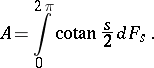Cayley transform

(diff) ← Older revision | Latest revision (diff) | Newer revision → (diff)
of a linear (dissipative) operatorwith domain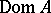dense in a Hilbert space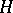The operator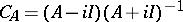, defined on the subspace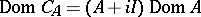. A matrix version of this transform was considered by A. Cayley. The Cayley transform establishes a correspondence between the properties of operatorswith spectra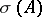"close" to the real line and operators with almost-unitary spectra (close to the circle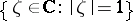). The following propositions are true: 1) ifis a linear dissipative operator, thenis a contraction (i.e.,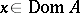) and; 2) ifis a contraction,and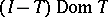is dense in, thenfor some linear dissipative operator; in fact,; 3)is symmetric (self-adjoint) if and only ifis isometric (unitary); 4)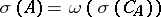, where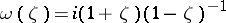, in particular,is bounded if and only if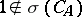; and 5) ifis an operator ideal in, then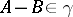implies; if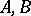are bounded operators, then the converse is also valid: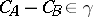implies. The Cayley transform also establishes a correspondence between certain other characteristics of the operatorsand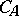: classifications of parts of the spectrum, multiplicities of spectra, structures of invariant subspaces, functional calculi, spectral decompositions, etc. Thus, ifis a self-adjoint operator with resolution of the identity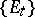,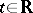, then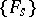,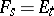for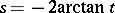, is a resolution of the identity forand